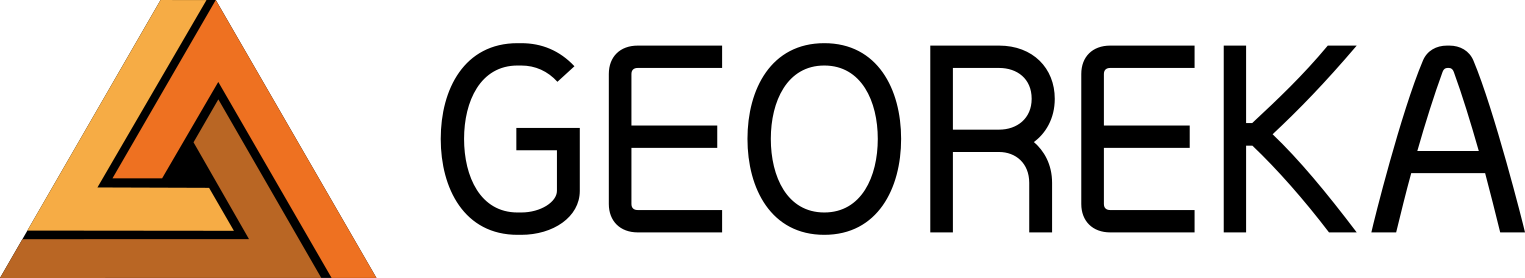#written by

Date

05/10/2018

## Modelling Stratigraphy with GEOREKA Software

Stratigraphic modelling can often be done directly from the data, and creating a geological model from stratigraphic drillhole data is therefore relatively straightforward. But although modelling stratigraphy in most cases is straightforward, in some cases it isn’t. If your project is fairly complex, contact us to demonstrate the more advanced tools.

Here, we will present a real data set in a simple setting without any faults to demonstrate basic concepts.

### Topography

The first step in most geological models is to define the topography. Generally, the topography is provided as a point cloud from something like a LIDAR scan, or as an existing triangulated surface. In some case however, there is no external data available. In that case, the collar points can be used to create the topography as in this example. Here we will take the collar points and convert them into indicator points. This is but one way to use the topography. Other methods are also possible, like creating a triangulated surface first.

The method we use in this example estimates the local curvature of the topography and uses that to create indicator points above and below the topography so that it can be converted to an implicit representation using a function descriptor like so:

### Overburden

The next step is to model the overburden. This can be modelled directly from the lithology data by creating an implicit representation from that.This means the intervals that are labelled as Overburden will be converted to positive indicator points. The rest as negative indicator points (outside Overburden or not Overburden). Since there are no negative points above the overburden the function will appear as a ‘flat’ surface separating the overburden from the unit below:

### Stratigraphic layers

Following the overburden, the actual stratigraphic layers can be modelled. For these, a similar approach is used by converting each layer to indicator points followed by creating an implicit function from them. However, one word of caution here. Using functional modelling in a naive way would create outside points for the units previously modelled as well. Each unit would become almost like an intrusive and is not ideal. Instead, indicator points for already modelled units need to be ignored (they have been modelled already). A way to think about it is that you are modelling the unit at the time it was created and means there were no other units above it. In GEOREKA we do this when we create the indicator points:

### Determine the relative ages

The approach described above implicitly assumes an order in which to model the units. This order depends on the relative age of each unit. Generally, younger units are modelled first. In GEOREKA software, whenever a unit is selected to be modelled, other units can be ignored, ie. no points are created for those ignored units.

As an example, to model the next youngest unit after the overburden, unit 7 is selected for the indicator points. Since overburden was already modelled in the first step, it is ignored here.

Similarly, for unit 6, the next after 7, both unit 7 and overburden are ignored. We repeat this process for all units down to unit 1, where units 2, 3, 4, 5, 6, 7 and the overburden are ignored.

### Complete stratigraphic model

When the domain functions for each stratigraphic layer are defined, they can be used to build the final model. Up until now, each function was not constrained by any other function. As a result, the functions describing units 1 to 7 appear to extend into the overburden or even beyond into space!

In our final step, a descriptor is set up to realize the complete stratigraphic model with all constraints in place. Again, the relative age determines the order in which each unit is defined in the model descriptor. Each domain (ie. each layer in this case) can only be defined where no previous domain exists. This means that in building the model, overburden will define the first layer. Where the overburden exists, units 1 to 7 can no longer be defined. Similarly, older units can not be defined where younger units exist.  The total model descriptor will look like this:

### End result

If the model descriptor is used to create a final stratigraphic model the result will look like this: